# Penalty functions, method of

(diff) ← Older revision | Latest revision (diff) | Newer revision → (diff)

A method for reducing constrained extremum problems to problems of unconstrained optimization. The method of penalty functions may be illustrated for problems in mathematical programming. Consider the problem of minimizing a function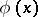on a set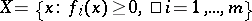in an-dimensional Euclidean space. A penalty function, or penalty (for violating the restrictions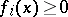,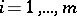), is a functiondepending onand a numerical parameterwith the following properties: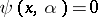ifand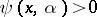if. Letbe any point where the function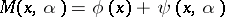takes an unconstrained (global) minimum, and let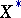be the set of solutions of the original problem. The function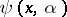is chosen such that the distance between the pointsand the settends to zero for, or, if it is not possible to ensure this condition, such that the following relation holds: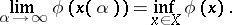Forone often chooses the function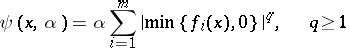(frequently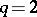).

The choice of a particular form for the functionis connected both with the problem of convergence of the method of penalty functions, and with problems arising in the unconstrained minimization of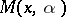.

A more general statement of the method of penalty functions is based on reducing the problem of minimization ofon a setto the problem of minimizing some parametric functionon a set of simpler structure (from the point of view of efficiency of applying numerical minimization methods) than the initial set.

The following well-known general result shows that the method of penalty functions is universal. Letandbe reflexive Banach spaces (cf. Reflexive space); let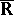be the extended real line; letbe a function defined onwith values inthat is weakly lower semi-continuous (cf. Semi-continuous function); let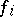,, be functions defined onwith values inthat are continuous in the weak topology of; let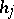,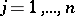, be functions defined on, with values in, that are continuous in the weak topologies of the spacesand(cf. Weak topology); and let the set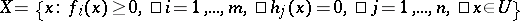be non-empty. Consider the problem of finding those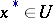for which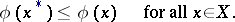(*)

For the functionwith,,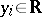,, consider the problem of finding those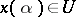and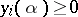,, for whichfor all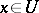,,. If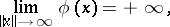then any weak limit point of an arbitrary sequence,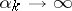,, is a solution of the problem (*) and, moreover,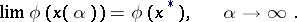How to Cite This Entry:
Penalty functions, method of. Encyclopedia of Mathematics. URL: http://encyclopediaofmath.org/index.php?title=Penalty_functions,_method_of&oldid=13425
This article was adapted from an original article by V.G. Karmanov (originator), which appeared in Encyclopedia of Mathematics - ISBN 1402006098. See original article